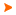Podaci o VU Početna stranicaArhiva nastavnih programaArhiva ak. kalendaraDynamics of Structural Systems
 Abbreviation: DIKOSU-Z Load: 30(L) + 0(E) + 15(LE) + 0(CE) + 0(PEE) + 0(FE) + 0(S) + 0(DE) + 0(P) + 0(FLE) + 0()
 Lecturers in charge: prof. dr. sc. Zdravko Terze Lecturers: doc. dr. sc. Dario Zlatar ( Lectures ) Viktor Pandža mag. ing. mech. ( Laboratory exercises ) Course description: Course objectives: Introduction to mechanical and mathematical modelling of structural systems with the aim of preparation of computational models for numeric analysis and dynamic simulation of aircraft structural systems.Enrolment requirements and required entry competences for the course: Mechanics II (Solids Kinematics and Dynamics)Student responsibilities: Students are required to regularly and actively participate in class and to accomplish the assigned tasks. The examination consists of written and oral parts. The final score is obtained as a result of written and oral exames.Grading and evaluation of student work over the course of instruction and at a final exam: Activities that are valued: written exam 50% oral exam 50%Methods of monitoring quality that ensure acquisition of exit competences: Continuity of students" work will be monitored through activities and exercises in the classroom and through individual and team execution of tasks. Acquired knowledge of students will be monitored through discussions during lectures and exercises. If necessary, the initial level of competence of students will be checked. The quality and success of the course will be monitored through the evaluation at the end of the semester, based on the success of the students at the final exam. Continuous selfevaluation will be done through the comparison of the instructional content of the course with the similar courses at EU universities. Also, the contacts with the professors at EU universities will be organized at regular basis, discussing relevant academic issues.Upon successful completion of the course, students will be able to (learning outcomes): enumerate elements of structural systems and characteristics of the kinematical and dynamical stuctural couplings understand and describe dynamical models for numerical simulation of aircraft structural systems based on discrete mechanicals models with kinematical constraints explain mathematical equations of scleronomic and rheonomic kinematical constraints of selected kinematical pairs and actuator elements of structural systems identify computational models of dynamics of multibody systems during analysis of numerical procedures for dynamic analysis and simulation of motion of aircrafts and spacecrafts as well as dynamic analysis of their mechanical subsystems identify and compare characteristics and definition parameters of mathematical models of multibody structural systems in minimal and descriptor form analyze basic numerical characteristics of computational procedures for dynamic simulation of aerospace strucutral systems with complex kinematic structureLectures 1. Dynamics of structural systems: numerical casestudies in aerospace engineering.2. Discrete mechanical systems and mechanical systems with distributed parameters: basic characteristics and structure of mathematical model.3. Introduction to mechanical modelling: objectives, specific tasks and characteristics. Basic approaches to mechanical modelling of structural multibody systems.4. Topological characteristics to the modelling. Degrees of freedom, coordinates of kinematical configuration.5. Discrete mechanical systems. NewtonEuler equations. Matrix form of dynamic equations of rigid body.6. Dynamic equations of "free" rigid body systems.7. Kinematical constraints. General form of kinematical constraints mathematical models.8. Basic mathematical structure of different kinamtical constraints. Scleronomic and rheonomic kinematical constraints, unilateral contacts.9. Dynamic equations of constrained multibody systems: introduction.10. Dynamic equations of constrained multibody systems: continuation. Minimal and descriptor form of mathematical model.11. Forward and inverse dynamics of mechanical system.12. Numerical methods in dynamics of structural multibody systems: ODE and DAE systems of differential equations, characteristics and basic principles of timeintegration.13. ODE and DAE systems of differential equations, characteristics and basic principles of timeintegration: continuation.14. Numerical examples, casestudies in aeronautical engineering: model of landing aircraft, landing gear dynamics.15. Presentation of different numerical casestudies in dynamics of aerospace structural systems: rotor dynamics, space vehicle structural system.Exercises 1. Dynamic analysis and simulation of structural systems: tasks and overview of different application cases.2. Discrete mechanical systems and mechanical systems with distributed parameters: numerical examples.3. Numerical casestudy assignment: dynamic simulation of the aircraft or space vehicle structural system / group based work.4. Mechanical model synthesis for assigned numerical case: objectives, assumptions, elements of discretisation.5. Definition of kinematical structure of the structural system.6. Mathematical modelling of kinematical constraints: introduction.7. Mathematical modelling of kinematical constraints: continuation.8. Synthesis of the system kinematical constraint matrix. The characteristics of system constraint matrix.9. Kinematical synthesis of the structural system mechanical model.10. Computational synthesis of the mathematical model of system dynamics: introduction.11. Computational synthesis of the mathematical model of system dynamics: continuation.12. Model preparation for the system dynamic simulation.13. Numerical integration of differential equations of motion: introduction. A choice of numerical method for timeintegration.14. Numerical integration of differential equations of motion: continuation. Numerical treatment of algebraic constraints. Results of dynamic simulation.15. Results of dynamic simulation. Interpretation of the results. Disscussion.
 Lecture languages: hr
 Compulsory literature: 1. Terze, Z.; Eiber, A. (University of Stuttgart), Introduction to Dynamics of Multibody Systems, FSB Zagreb, e-book, 2002. 2. Haug, E.: Computer-Aided Kinematics and Dynamics of Mechanical Systems, Allyn and Bacon, 1989.
 Recommended literature: 3. Shabana, A. A.: Dynamics of multibody systems, Wiley, New York, 1989.
 Prerequisit for enrollment: Completed : Mechanics IIILegend L - Lectures FLE - Practical foreign language exercises - E - Exercises LE - Laboratory exercises CE - Project laboratory PEE - Physical education excercises FE - Field exercises S - Seminar DE - Design exercises P - Practicum * - Not graded| Početna stranica | Visoka učilišta | Korisničke stranice | | Na vrh | Copyright (c) 2006. Ministarstva znanosti, obrazovanja i športa. Sva prava zadržana. Programska podrška (c) 2006. Fakultet elektrotehnike i računarstva. Oblikovanje(c) 2006. Listopad Web Studio. Posljednja izmjena 2019-06-07